Next: Exercises Up: Gravitational Potential Theory Previous: Perihelion Precession of the

# Perihelion Precession of Mercury

If the calculation described in the previous section is carried out more accurately, taking into account the slight eccentricities of the planetary orbits, as well as their small mutual inclinations, and retaining many more terms in the expansions (1015) and (1017), then the perihelion precession rate of the planet Mercury is found to be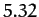arc seconds per year. However, the observed precession rate is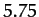arc seconds per year. It turns out that the cause of this discrepancy is the general relativistic correction to Newtonian gravity.

General relativity gives rise to a small correction to the force per unit mass exerted by the Sun (mass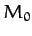) on a planet in a circular orbit of radius, and angular momentum per unit mass. In fact, the modified formula for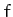is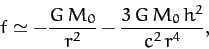(1025)

whereis the velocity of light in vacuum. It follows that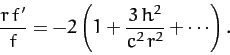(1026)

Hence, from Equation (317), the apsidal angle is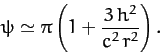(1027)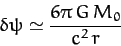(1028)
radians per revolution due to the general relativistic correction to Newtonian gravity. Here, use has been made of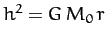. It follows that the rate of perihelion precession due to the general relativistic correction is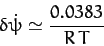(1029)
arc seconds per year, whereis the mean orbital radius in mean Earth orbital radii, and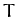is the orbital period in years. Hence, from Table 1, the general relativistic contribution to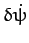for Mercury isarc seconds per year. It is easily demonstrated that the corresponding contribution is negligible for the other planets in the Solar System. If the above calculation is carried out sightly more accurately, taking the eccentricity of Mercury's orbit into account, then the general relativistic contribution tobecomesarc seconds per year. It follows that the total perihelion precession rate for Mercury is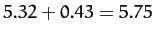arc seconds per year. This is in exact agreement with the observed precession rate. Indeed, the ability of general relativity to explain the discrepancy between the observed perihelion precession rate of Mercury, and that calculated from Newtonian dynamics, was one of the first major successes of this theory.